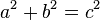# Simple wikipedia

This gives simple explanations for things like Pythagoras’ Theorem.

In mathematics, the Pythagorean theorem or Pythagoras’ theorem is a statement about the sides of a right triangle.

One of the angles of a right triangle is always equal to 90 degrees. This angle is the right angle. The two sides next to the right angle are called the legs and the other side is called the hypotenuse. The hypotenuse is the side opposite to the right angle, and it is always the longest side.

The Pythagorean theorem says that the area of a square on the hypotenuse is equal to the sum of the areas of the squares on the legs. In the picture below, the area of the blue square added to the area of the red square makes the area of the purple square. It was named after the Greek mathematician Pythagoras:

If the lengths of the legs are a and b, and the length of the hypotenuse is c, then,$a^2+b^2=c^2$.